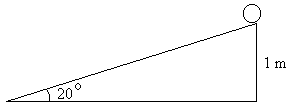First Name: ___________________ Last Name: ____________________ Section: _________

October 29, 1999 Physics 207

Exam 2

Print your name and section clearly on all five pages. (If you do not know your section number, write your TAs name.) Show all work in the space immediately below each problem. Your final answer must be placed in the box provided. Problems will be graded on reasoning and intermediate steps as well as on the final answer. Be sure to include units wherever necessary, and the direction of vectors. Each problem is worth 25 points. In doing the problems, try to be neat. Check your answers to see that they have the correct dimensions (units) and are the right order of magnitudes. You are allowed one 8½ x 11" sheet of notes and no other references. The exam lasts exactly 50 minutes.

(Do not write below)

SCORE:

Problem 1: __________

Problem 2: __________

Problem 3: __________

Problem 4: __________

TOTAL: ___________

First Name: ___________________ Last Name: ____________________ Section: _________

1. An automobile traveling 40 m/s goes around a curve with a radius of 200 m.

a. What is the acceleration of the automobile? (5 pts.)

b. If the road is not banked, what coefficient of friction is required to keep the automobile from sliding across the road? (5 pts.)

c. At what angle must the road bank to prevent sliding in the absence of friction at this speed? (5 pts.)

d. At the angle calculated above, what coefficient of friction is required to keep a parked car from sliding off the road? (5 pts.)

e. If the moving automobile consumes 50 horsepower (37.3 kW), what is the drag force acting on it? (5 pts.)

First Name: ___________________ Last Name: ____________________ Section: _________

2. A 50-g mass is launched vertically upward by a spring-loaded gun having a spring with k = 8000 N/m compressed 8 cm from its equilibrium length.

a. What force is required to compress the spring? (5 pts.)

b. What is the work done on the mass by the spring? (5 pts.)

c. With what speed is the mass moving when it leaves the gun? (5 pts.)

d. What is the impulse of the spring on the mass? (5 pts.)

e. How high does the mass go? (5 pts.)

First Name: ___________________ Last Name: ____________________ Section: _________

3. 1200-kg car moving at 15 m/s collides head-on with a 2000-kg truck initially at rest and sticks to the truck after the collision.

a. What is their velocity just after the collision? (5 pts.)

b. What is the impulse on the car? (5 pts.)

c. If the collision lasts 0.5 s, what is the average deceleration of the car? (5 pts.)

d. If the collision lasts 0.5 s, what is the average force on the car? (5 pts.)

e. What fraction of the initial energy is lost in the collision? (5 pts.)

First Name: ___________________ Last Name: ____________________ Section: _________

4. A uniform solid cylinder starts from rest at a height of 1 m and rolls without slipping down a plane inclined at an angle of 20° from the horizontal as shown.a. What is the speed of its center of mass when it reaches the bottom of the incline? (9 pts.)

b. What is the magnitude of the acceleration of its center of mass? (8 pts.)

c. What minimum coefficient of friction is required to prevent the cylinder from slipping? (8 pts.)